# splay详解（一）

## 前言

Spaly是基于二叉查找树实现的，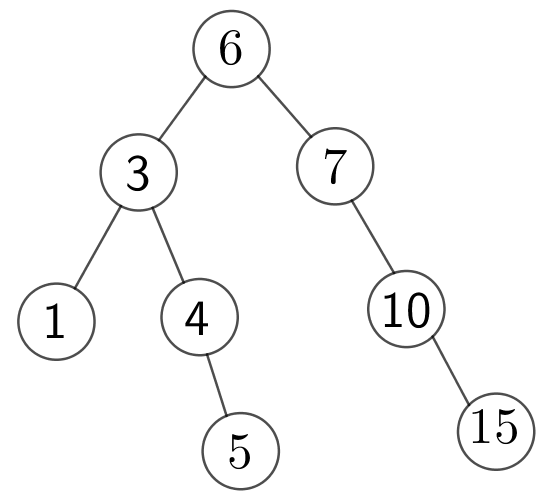## Splay简介

Splay是平衡树的一种，中文名为伸展树，由丹尼尔·斯立特Daniel Sleator和罗伯特·恩卓·塔扬Robert Endre Tarjan在1985年发明的(mmp怎么又是tarjan)

• 什么样的点是查找频率高的点？

•  怎么实现把节点搬到根这种操作？

## Splay基本操作

### rotate

#### 情况1B与X，X与Y，X与R

B会成为Y的左儿子

Y会成为X的右儿子

X会成为R的儿子，具体是什么儿子，这个要看Y是R的啥儿子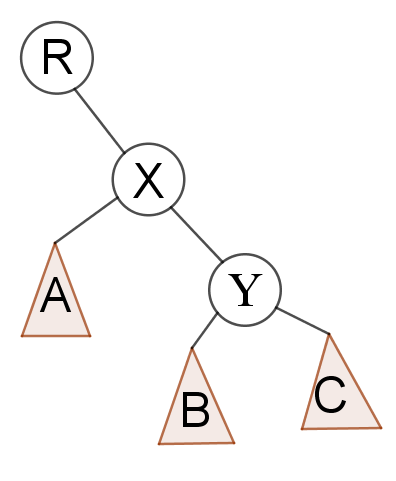#### 情况2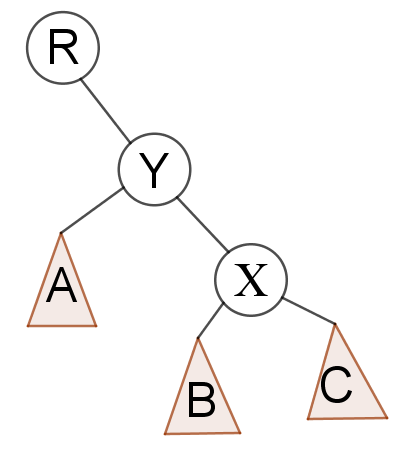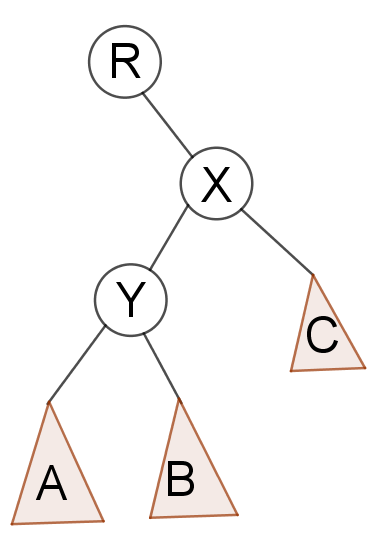bool ident(int x) {
return tree[tree[x].fa].ch == x ? 0 : 1;
}

int Y = tree[x].fa;
int R = tree[Y].fa;
int Yson = ident(x); //x是y的哪个孩子
int Rson = ident(Y);

B的情况我们可以根据X的情况推算出来，根据^运算的性质，0^1=1,1^1=0,2^1=3,3^1=2，而且B相对于X的位置一定是与X相对于Y的位置是相反的

（否则在旋转的过程中不会对B产生影响）

int B = tree[x].ch[Yson ^ 1];

B成为Y的哪个儿子与X是Y的哪个儿子是一样的

Y成为X的哪个儿子与X是Y的哪个儿子相反

X成为R的哪个儿子与Y是R的哪个儿子相同

connect(B, Y, Yson);
connect(Y, x, Yson ^ 1);
connect(x, R, Rson);

connect函数这么写，挺显然的

void connect(int x, int fa, int how) { //x节点将成为fa节点的how孩子
tree[x].fa = fa;
tree[fa].ch[how] = x;
}

### Splay

Splay(x,to)是实现把x节点搬到to节点1.to是x的爸爸，

update in 2018.2.19

if (tree[tree[x].fa].fa == to) rotate(x);

2.x和他爸爸和他爸爸的爸爸在一条线上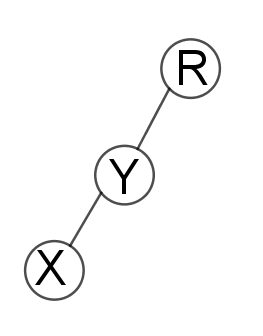else if (ident(x) == ident(tree[x].fa)) rotate(tree[x].fa), rotate(x);

3.x和他爸爸和他爸爸的爸爸不在一条线上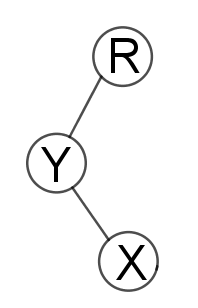void splay(int x, int to) {
to = tree[to].fa;
while (tree[x].fa != to) {
if (tree[tree[x].fa].fa == to) rotate(x);
else if (ident(x) == ident(tree[x].fa)) rotate(tree[x].fa), rotate(x);
else rotate(x), rotate(x);
}
}

## 后记

posted @ 2017-11-25 20:36  自为风月马前卒  阅读(15502)  评论(14编辑  收藏  举报